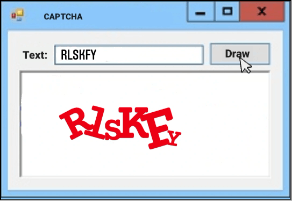C# Program to generate CAPTCHA (random string)

CAPTCHA  word is made by the term ‘Completely Automated Public Turing Test’ It is a program that used to give some kind of access to humans which shouldn’t be given to any machine or a robot. It is some type of generated test.

CAPTCHA reduces hackers, spam attackers, and fake visitors or entries from websites. This test most people can easily pass but a computer program cannot. The term CAPTCHA was discovered in 2000 by Luis von Ahn, Manuel Blum, Nicholas Hopper, and John Langford of Carnegie Mellon University. These 4 have been credited with creating the first CAPTCHA, which was used by Yahoo!

Modern text-based CAPTCHAs are designed with three separate abilities that require these are-

• Invariant recognition
• segmentation
• parsing

They have used to correctly complete the task with any consistency.

Invariant recognition

Ability to identify a large amount of variation in the shapes of letters is called invariant recognition.

Segmentation

We can say that the ability to separate one letter from another, is also make CAPTCHA’s difficult, it is called segmentation. Because characters are gambled together with no white space in between.

Parsing

Context is also critical. The CAPTCHA must be understood whole to be correctly identify each character.
Each of these problems creates a challenge for a computer. By the presence of all three at the same time can make the CAPTCHAs so difficult to solve. Humans can do this type of task but the computer doesn’t. While segmentation and recognition are two separate processes for understanding an image for a computer, but for a person, they are part of the same process.

Program to generate CAPTCHA(random string) Image in C#

private Random Rand = new Random();

// Make a captcha image for the text.
int min_size, int max_size, int wid, int hgt)
{
// Make the bitmap and associated Graphics object.
Bitmap bm = new Bitmap(wid, hgt);
using (Graphics gr = Graphics.FromImage(bm))
{
gr.SmoothingMode = SmoothingMode.HighQuality;
gr.Clear(Color.White);

// See how much room is available for each character.
int ch_wid = (int)(wid / txt.Length);

// Draw each character.
for (int i = 0; i < txt.Length; i++)
{
float font_size = Rand.Next(min_size, max_size);
using (Font the_font = new Font("Times New Roman",
font_size, FontStyle.Bold))
{
DrawCharacter(txt.Substring(i, 1), gr,
the_font, i * ch_wid, ch_wid, wid, hgt);
}
}
}

return bm;
}

The method creates a Bitmap of the desired size, and sets its SmoothingMode property, and clears it. After that then it calculates the width it can allow each character. Then the code loops over the message characters. For each one character, it generates a random font size and creates a font of that size. In the end the code calls the following DrawCharacter method to draw the character.

// Draw a deformed character at this position.
private int PreviousAngle = 0;
private void DrawCharacter(string txt, Graphics gr,
Font the_font, int X, int ch_wid, int wid, int hgt)
{
// Center the text.
using (StringFormat string_format = new StringFormat())
{
string_format.Alignment = StringAlignment.Center;
string_format.LineAlignment = StringAlignment.Center;
RectangleF rectf = new RectangleF(X, 0, ch_wid, hgt);

// Convert the text into a path.
using (GraphicsPath graphics_path = new GraphicsPath())
{
the_font.FontFamily, (int)(Font.Style),
the_font.Size, rectf, string_format);

// Make random warping parameters.
float x1 = (float)(X + Rand.Next(ch_wid) / 2);
float y1 = (float)(Rand.Next(hgt) / 2);
float x2 = (float)(X + ch_wid / 2 +
Rand.Next(ch_wid) / 2);
float y2 = (float)(hgt / 2 + Rand.Next(hgt) / 2);
PointF[] pts = {
new PointF(
(float)(X + Rand.Next(ch_wid) / 4),
(float)(Rand.Next(hgt) / 4)),
new PointF(
(float)(X + ch_wid - Rand.Next(ch_wid) / 4),
(float)(Rand.Next(hgt) / 4)),
new PointF(
(float)(X + Rand.Next(ch_wid) / 4),
(float)(hgt - Rand.Next(hgt) / 4)),
new PointF(
(float)(X + ch_wid - Rand.Next(ch_wid) / 4),
(float)(hgt - Rand.Next(hgt) / 4))
};
Matrix mat = new Matrix();
graphics_path.Warp(pts, rectf, mat,
WarpMode.Perspective, 0);

// Rotate a bit randomly.
float dx = (float)(X + ch_wid / 2);
float dy = (float)(hgt / 2);
gr.TranslateTransform(-dx, -dy, MatrixOrder.Append);
int angle = PreviousAngle;
do
{
angle = Rand.Next(-30, 30);
} while (Math.Abs(angle - PreviousAngle) < 20);
PreviousAngle = angle;
gr.RotateTransform(angle, MatrixOrder.Append);
gr.TranslateTransform(dx, dy, MatrixOrder.Append);

// Draw the text.
gr.FillPath(Brushes.Red, graphics_path);
gr.ResetTransform();
}
}
}

The method makes a StringFormat object to center a character in a rectangle. Then it builds the rectangle that will hold the character.

After that code creates a GraphicsPath object and adds a character to it in the proper position then randomly picks some points in the character’s area and uses the GraphicsPath object’s Warp method to warp the character’s bounding rectangle into those points, for distorting the character’s image.

After that, the code applies a transformation to the Graphics object to rotate the character around its center by a random angle.

Because in tests, I was seeing a lot of characters with similar rotations, so here I added a static variable and a loop to ensure that each character’s rotation differs by the rotation of the previous character at least 20 degrees.

In the end, the subroutine draws the warped and rotated character onto the Graphics object.

Output screenSo in this way, we can generate a random string or CAPTCHA image in C# WinForms.